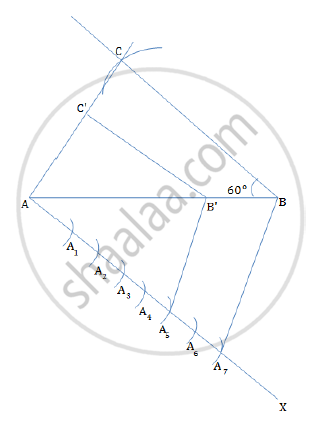# Construct a triangle ABC in which AB = 5 cm, BC = 6 cm and ∠ABC = 60˚. Now construct another triangle whose sides are 5/7 times the corresponding sides of ΔABC. - Mathematics

Construct a triangle ABC in which AB = 5 cm, BC = 6 cm and ∠ABC = 60˚. Now construct another triangle whose sides are 5/7 times the corresponding sides of ΔABC.

#### Solution

Steps for construction:
1. Draw a line segment AB = 5 cm.
2. At B construct m∠ABC = 60°
3. Take a measure of 6 cm, and draw an arc from B on BC.
4. Join AC to obtain ΔABC.
5. Below AB, make an acute angle ∠BAX.

6. Since 7 > 5, mark off 7 points A1, A2, A3, A4, A5, A6 and A7 such that  AA1 = A1A2 = A2A3 = A3A4 = A4A5 = A5A6 = A6A7.
7. Join A7B.
8. Since we have to construct a triangle each of whose sides is 5/7 of the corresponding sides of ABC. So take five parts out of seven equal parts on AX. i.e. from point A5,      draw A5B' || A7B, meeting AB at B'.
9. From B', draw B'C' || BC, meeting AC at C'
10.ΔAB'C' is the required, each of the sides is five-seventh of the corresponding sides of ΔABC.Concept: Division of a Line Segment
Is there an error in this question or solution?
2014-2015 (March) All India Set 3

Share